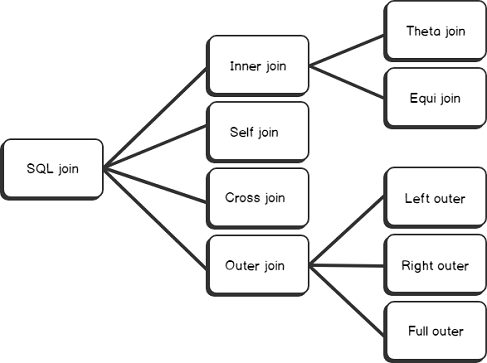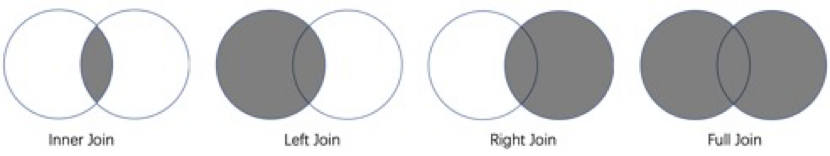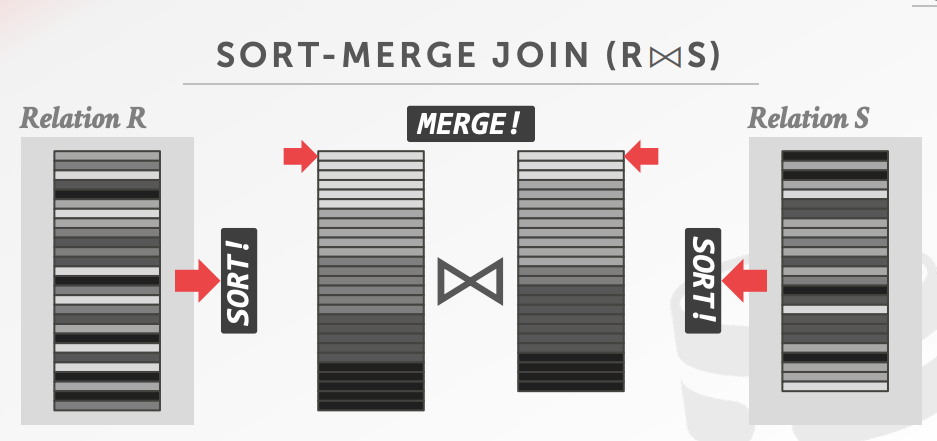# Community

Blog Events Webinars Tutorials Forum
×
Community Blog How to Write a High-Performance SQL Join: Implementation and Best Practices of Joins

# How to Write a High-Performance SQL Join: Implementation and Best Practices of Joins

This article aims to sort the usage and internal implementation of joins.

By Yunlei, from Alibaba Cloud Storage

Join is one of the most common concepts in databases and data warehouses. In data models of relational databases, different data is often placed in different tables to avoid redundant data storage, which are divided into fact tables and dimension tables. This can save data storage space. However, you need to associate multiple tables through joins when analyzing data. Joins are indispensable for data analysis. Joins have many types and different ways of usage and implementation. Different ways of usage and implementation will cause many differences in performance. This article aims to thoroughly sort the usage and internal implementation of joins. In addition, it aims to help you understand how to write a high-performance SQL Join by learning its internal implementation.

## Types of JoinsIn general, SQL Joins are classified into Inner Join, Outer Join, Self Join, and Cross Join.### Inner Join

Inner Join is one of the most frequently used joins. The left and right tables are joined by predicates. Only the rows that appear in both the left table and the right table meet the condition. It means the rows are in the set intersection of the left and right tables. The syntax is `select A.x, B.y from A join B on A.x = B.y`. Inner Join does not distinguish the order of the left and right tables. A inner join B is equivalent to B join A.

Inner Join is divided into Equal Join and Non Equal Join (Theta Join). The difference is that Equal Join is a certain field in the left table that is equal to a field in the right table under the join condition. The join condition of Theta Join is not the equality condition but may be greater-than or less-than conditions.

## Outer Join

Outer Join includes Left Join, Right Join, and Full Join. Please refer to the figure above for the different points of each join.

Left Join returns all rows in the left table, regardless of whether these rows match the right table. The data is divided into two categories: the data that matches the right table and the data that does not match the right table. For the data in the intersection of the left and right tables that matches the right table, the columns of the left and right tables are output respectively. For the data that is not in the intersection nor does not match the right table, the column value of the left table is output, and the column value of the right table is null. Left Join does not allow the Interchanging of the left and right tables.

Right Join and Left Join are symmetrical. It returns all rows of the right table, regardless of whether these data rows match the left table. These data are divided into two categories: the data that matches the left table and the data that does not match the left table. For the data in the intersection of the left and right tables that matches the left table, the columns of the left and right tables are output. For rows that are not in the intersection, the columns of the right table are normally output, while the columns of the left table are null. Right Join does not allow the Interchanging of the left and right tables. However, Left Join and Right Join are symmetrical. Therefore, a Left Join can be converted into a Right Join. `A left join B` is equivalent to `A right join B`.

Full join is a combination of Left Join and Right Join, which returns the set union of the left and right tables. The result of Full Join contains three parts of data: the data in the set intersection of the left and right tables (in which the data matches the left and right tables at the same time), the data only matching the left table, and the data only matching the right table. For the data in the set intersection of the left and right tables, the column values of the left and right tables are output. For the data only matching the left table, the columns of the left table are output, while the columns of the right table are null. For the data only matching the right table, the columns of the right table are output, while the columns of the left table are null.

## Differences between Inner Join and Outer Join

The difference between Inner Join and Outer Join is that the result of Inner Join is the rows that both exist in the left and right tables. Thus, the result is the set intersection of the two tables and inside the left and right tables. The result of Outer Join may contain rows that do not belong to the table, such as Left Join, Right Join, and Full Join. Some results of Outer Join belong to the outside of this table, so it is called Outer Join.

## Cross Join

Cross Join is the Cartesian product of two tables, which indicates the N*M types of combination of the left and right tables. Cross Join is rarely used because not all combinations are meaningful. Generally, the filtering criteria are attached to combinations to select meaningful results. Here is the use method: `A cross join`.

## Self Join

Self Join means the table is joined with itself. The left table is the same as the right table. It could be inner join or outer join.

## Semi Join

In Semi Join, rows returning from one table perform an incomplete join query with data rows of another table. If matched data rows are found, the Semi Join returns results and stops the search. Typical queries include `in` and `exists`.

## Anti Semi Join

In Anti-semi-join, rows returning from one table perform an incomplete join query with data rows of another table. Then, the Anti Semi Join returns unmatched data. Typical queries are `not exists` and `not in`.

For example,

``select * from A where not exists (select B.y from B)``

## Join Implementation

Understanding the system implementation helps you write SQL statements with the best performance. How do you implement a simple hash algorithm without any optimization? Use a two-layer loop to traverse each row of the left and right tables in turn and then determine the join condition. If a row complies with the join condition, the row is output. This practice is called Product Join.

``````for rowX in left_table:
for rowY in right_table:
if rowX match rowY
output rowX and rowY``````

This practice can achieve the goal, but it is clear that the time complexity of this approach is O（N*M), and the speed is very slow. Thus, there are several faster implementation methods (listed below).

### Sort Merge

First, it sorts the left and right tables and then merges the two sorted tables according to the K-way merging algorithm. The time complexity of sorting is O(nlog(n)), and the merging time complexity is O(n). The overall time complexity is O(nlog(n)).### Hash Join

The algorithm of Hash Join is to build a hash table for the right table. Then, traverse the left table and probe the hash table based on the hash value of the join key. Therefore, the right table is called the build side, and the left table is called the probe side.

The time complexity of building a hash table is O(n). The time complexity of probing is also O(n). More importantly, Hash Join can be used for distributed joins. When the amount of data is too large, you can hash data to different machines, and hash the same data to the same machine for matching. Distributed machines can be used to solve the join problem of big data.

Hash Join requires that both the left and right tables calculate hash values and then distribute data to other machines based on the hash key to execute the join. If the data is large, shuffle will cost a lot. At this time, here are two situations. If the other table is also very large, you can only do distributed processing by using hashing. However, if the other table is very small, you can directly copy the small table broadcast to the machine where the large table is located, thus avoiding shuffle.

### Shuffle Sort Merge Join

You can also use Shuffle Sort Merge Join in addition to Shuffle Hash Join for joining large tables. The difference is that Hash Join is shuffled to a fixed machine according to a specific hash key. However, Shuffle Sort Merge Join can be shuffled to a fixed machine according to a broader partition key. The data of the same partition is shuffled to the same machine and then joined according to the sort merge algorithm of the single machine.

### Different Approaches between Relational Databases and Data Warehouses

There is an implicit premise for the implementations of join mentioned above that the data exists in the data warehouse. The amount of data is relatively large and is stored in multiple partitions. The left and right tables are stored in different machines. However, all the data of the left and right tables are stored in the same machine in a single-machine relational database. Therefore, the algorithms of the two vary wildly. It is natural for data warehouses to shuffle data and move the left and right tables to the same machine. However, there are different schemes for optimization, depending on the size of the tables. If a table is very small, it is only required to broadcast the small table. If both tables are large, it is required to shuffle two tables.

### Equal Join and None Equal Join

If all the join conditions are equality conditions, during the joins, you can directly shuffle according to the join conditions and build a hash table according to the hash key at the same time. Therefore, when probing the hash table, you can use the hash table to search for data at the O(1) level.

However, if the join conditions contain non-equality conditions (or contain the or condition), you need to verify conditions line by line during the joins.

## Best Practices

This article introduces the usage and internal implementation of SQL. We can roughly understand how to write a high-performance Join statement by understanding the internal implementation.

1. Let the large table join the small table rather than the large table joining the large table.
2. Use the equality condition and the and condition in the join conditions, but do not use the or condition.
3. Use Inner Join or Outer Join instead of Cross Join.
1 2 1
Share on

# Related Products

• ##Hologres

A real-time data warehouse for serving and analytics which is compatible with PostgreSQL.

• ##Personalized Content Recommendation Solution

Help media companies build a discovery service for their customers to find the most appropriate content.